## Outline

• Themes: control presentation of non-data elements
• Saving your work: to include in reports, presentations, etc.

## Visual Appearance

So far have mostly discussed how to get the data displayed the way you want, focusing on the essence of the plot.

Themes give you a huge amount of control over the appearance of the plot, the choice of background colours, fonts and so on.

## Built-In Themes

The default grey theme:

qplot(Total.Assists, Total.Rebounds, data = nba)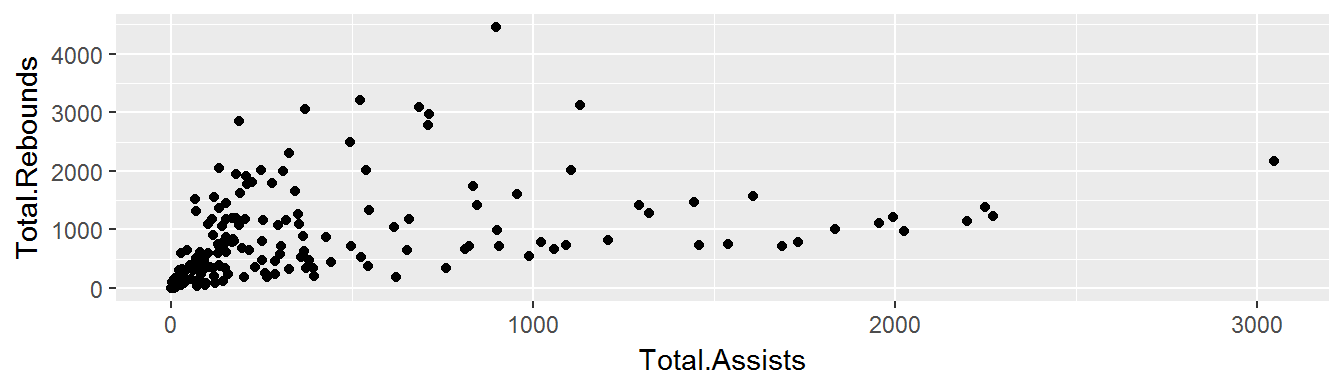A theme with white background:

qplot(Total.Assists, Total.Rebounds, data = nba) + theme_bw()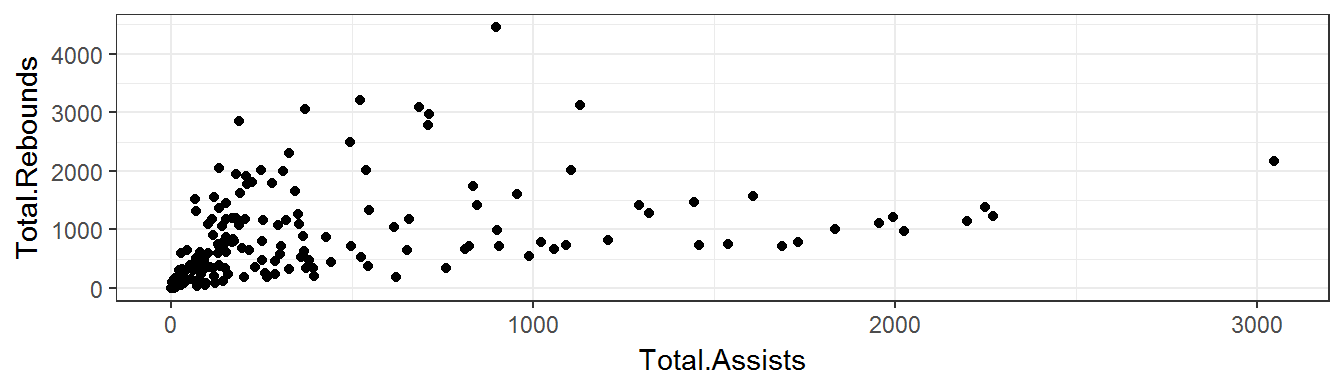Printing out the statements below is the best way of seeing all the default options.

theme_bw()
theme_grey()

## Plot Title

You can change the title for an individual plot with

labs(title = "My title")
#or
ggtitle("My title")
qplot(Total.Assists, Total.Rebounds, data = nba) +
ggtitle("Assists vs Rebounds in NBA")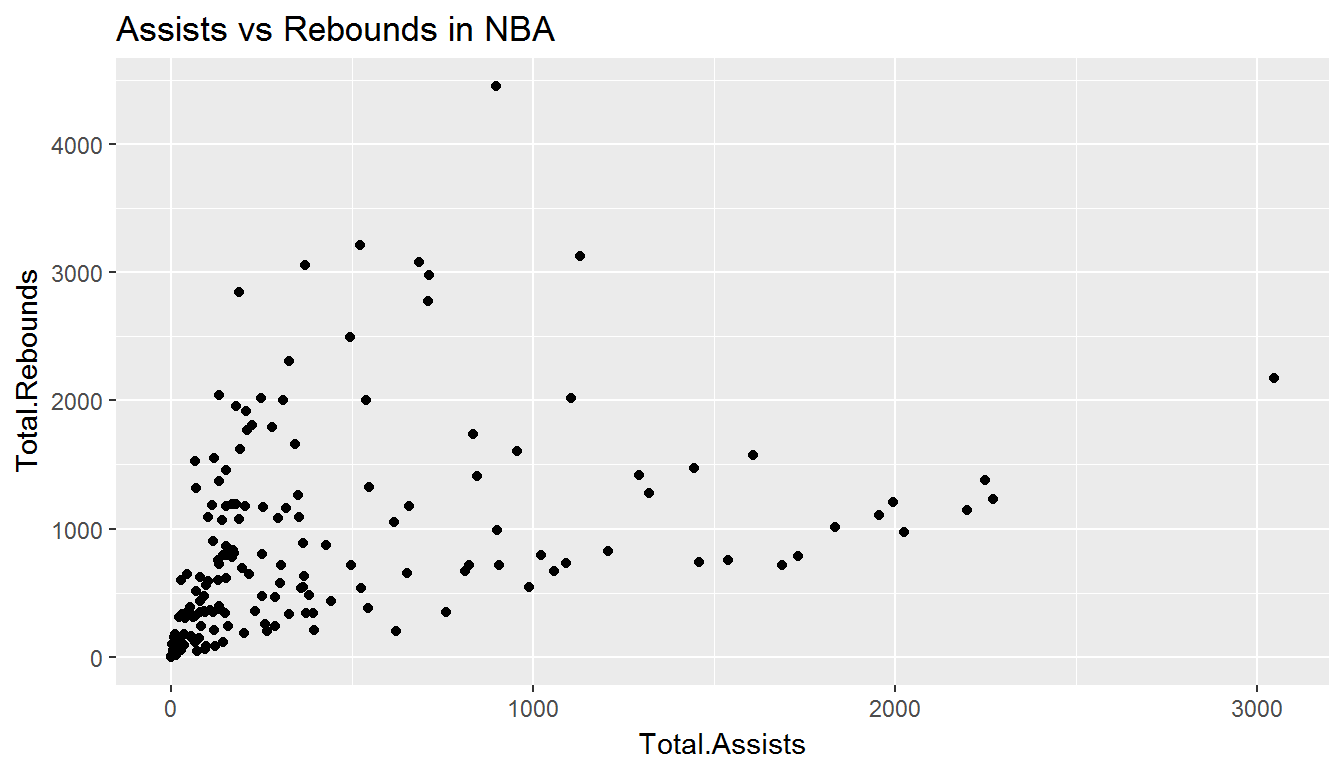qplot(Total.Assists, Total.Rebounds, data = nba) +
labs(title = "Assists vs Rebounds in NBA")## Elements

You can make your own theme, or modify an existing one.

Themes are made up of elements which can be one of:

• element_line()
• element_text()
• element_rect()
• element_blank()

This gives you a lot of control over plot appearance.

## Elements we can set

• Axis: axis.line, axis.text.x, axis.text.y, axis.ticks, axis.title.x, axis.title.y
• Legend: legend.background, legend.key, legend.text, legend.title
• Panel: panel.background, panel.border, panel.grid.major, panel.grid.minor
• Strip: strip.background, strip.text.x, strip.text.y

## Modifying a plot

There are many parameters we can change!

p <- qplot(Total.Assists, Total.Rebounds, data = nba) +
ggtitle("Total Rebounds vs. Total Assists")
p + theme(plot.title = element_text(hjust = .5,colour = "orange", face = "bold"))+
scale_x_continuous(
limits = c(0,4000), breaks = seq(0,4000,500)) # Changes the scale of the x axis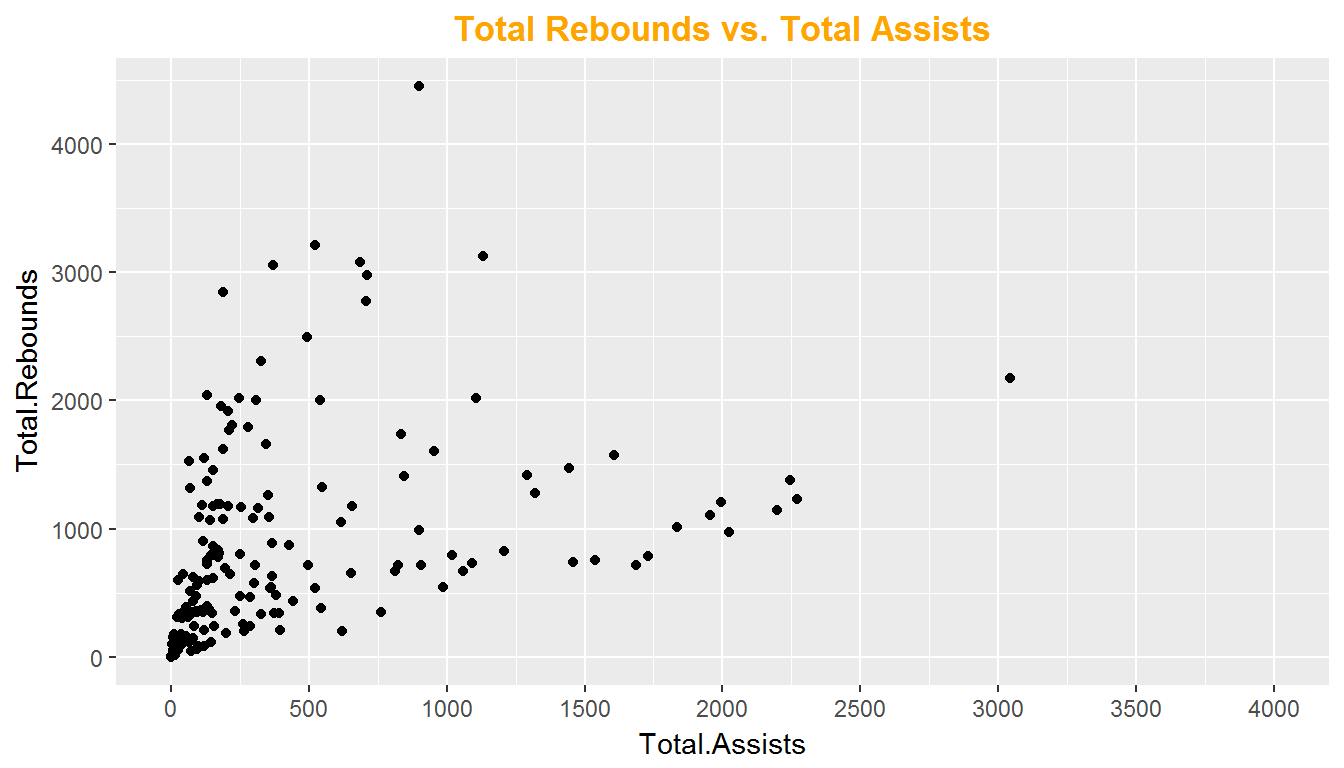## Removing Axes

We could also choose to remove all axes (helpful for maps):

p + theme(
axis.text.x = element_blank(),
axis.text.y = element_blank(),
axis.title.x = element_blank(),
axis.title.y = element_blank(),
axis.ticks.length = unit(0, "cm")
)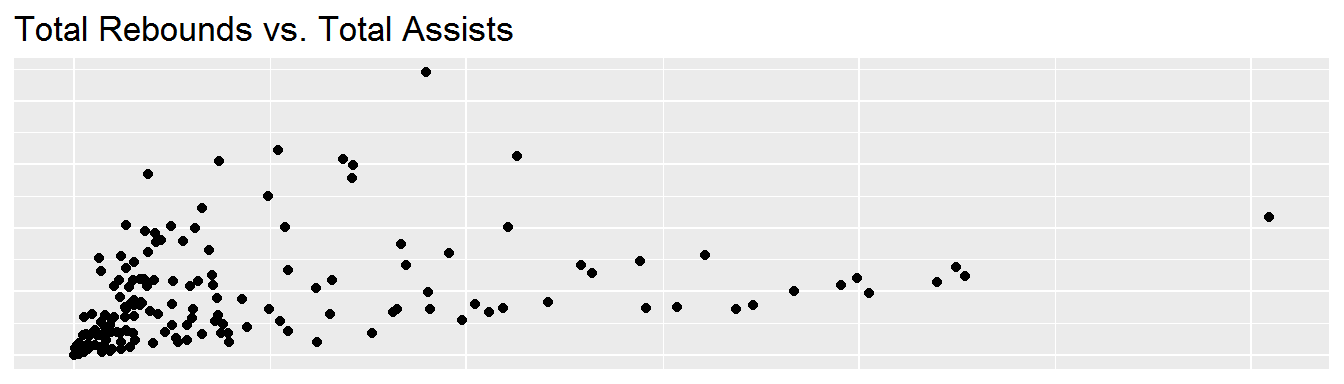One should experiment by removing one parameter at a time to understand what each one does.

## Relabeling Axes

You can rename axis titles by adding:

p + labs(x = "Total Assists", y = "Total Rebounds")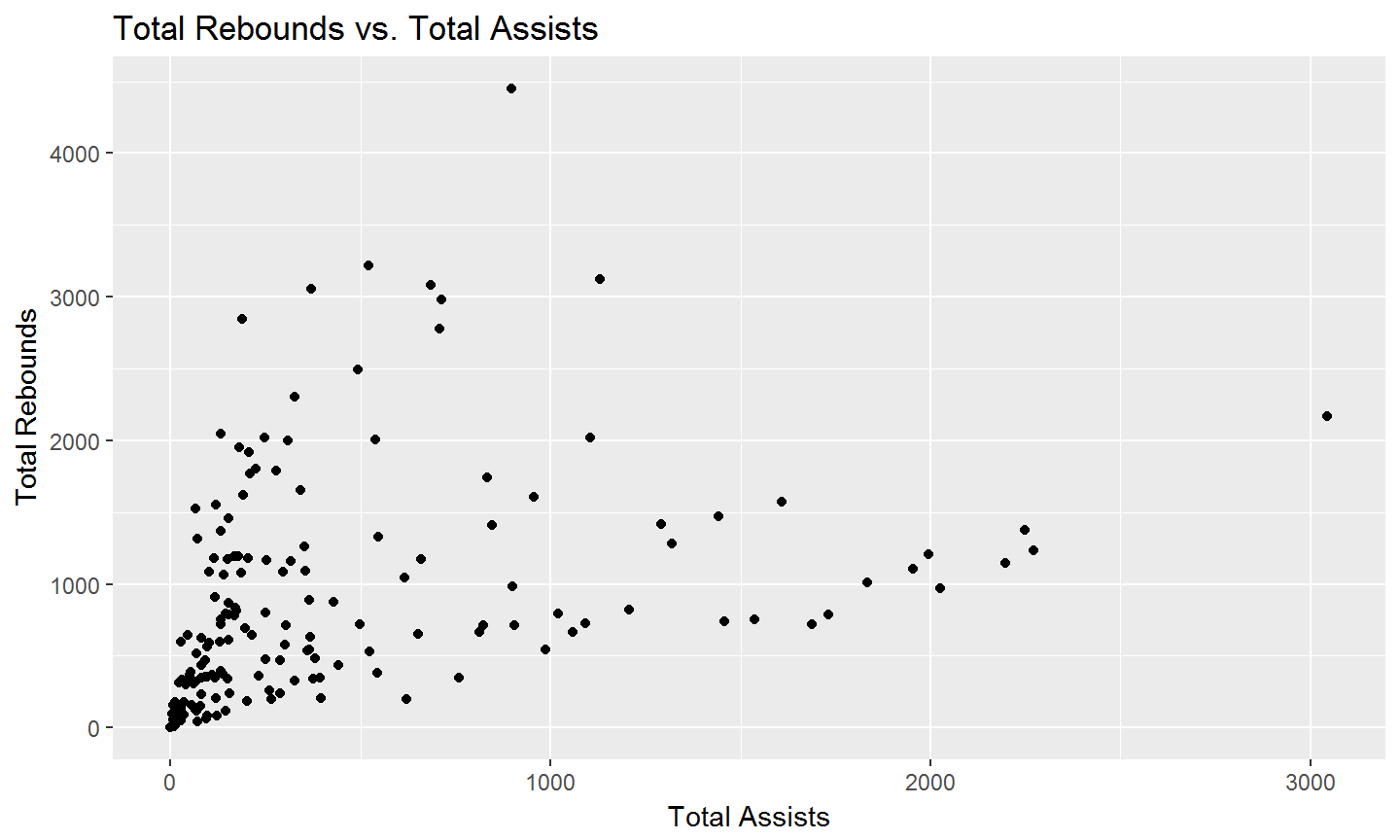The ggsave() function will automatically save the last plot produced:

qplot(Total.Assists, Total.Rebounds, data = nba)
ggsave("nba.png")
ggsave("nba.pdf")
ggsave("nba.png", width = 6, height = 6)

We can also explicitly tell it which plot to save:

dplot <- qplot(Total.Assists, Total.Rebounds, data = nba)
ggsave("nba.png", plot = dplot, dpi = 72)

## Your

1. Save a pdf of a scatterplot of Points Per Game vs. Field Goal Percentage such that:
• The title is blue and italic
• The background is white
• The points are colored orange
2. Save a png of the same scatterplot.

plot <- qplot(Field.Goal.Percentage, Points.Per.Game, data = nba) +
geom_point(aes(colour = "orange"),show.legend = FALSE)
ggsave("nba.png", plot = plot, dpi = 72)